## 椭圆曲线加密与NSA后门考古

2020-06-05 18:52:29  [ 以太坊 ]      留言：0      阅读：18

2020-05-17
12024

# 椭圆曲线

`y^2 = x^3 + a*x + b`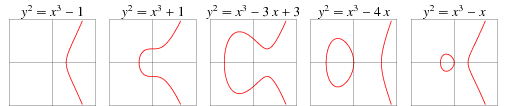## 实数集

1. 封闭性：若a和b属于群G，则a+b也属于群G
2. 结合性：(a+b)+c = a+(b+c)
3. 存在单位元θ，使得a + θ = θ + a = a
4. 群中每个元素都存在逆元素，即对于任意元素a存在b，使得a + b = θ

• 交换律：a + b = b + a

• 群的元素是椭圆曲线上的点
• 单位元是无穷远点0
• 点P的逆是它关于x轴的对称点
• 加法的定义为：对于三个同一直线上的非零点P、Q和R，它们的和为P+Q+R =0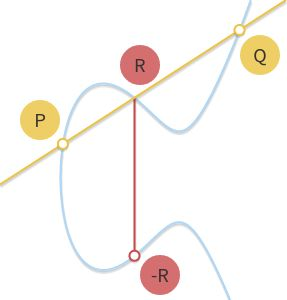```# 直线斜率为m
m = (Py - Pq) / (Px - Qx)
Rx = m^2 - Px - Qx
Ry = Py + m(Rx - Px)```

`n * P = P + P + P + ... + P`

`151 * P = 2^4 * P + 2^2 * P + 2^1 * P + 2^0 * P`

## 有限域

GF(p)元素集合为所有从0p-1的整数，其加法和乘法可以转换为模运算，也称为时钟算术，例如对GF(23)：

• 加法：(18+9) mod 23 = 4
• 减法：(7-14) mod 23 = 16
• 乘法：4 * 7 mod 23 = 5
• 加法逆元：-5 mod 32 = 18
• (5 + (-5)) mod 23 = (5 + 18) mod 23 = 0
• 乘法逆元：9^-1 mod 23 = 18
• 9 * 9^-1 mod 23 = (9 * 18) mod 23 = 1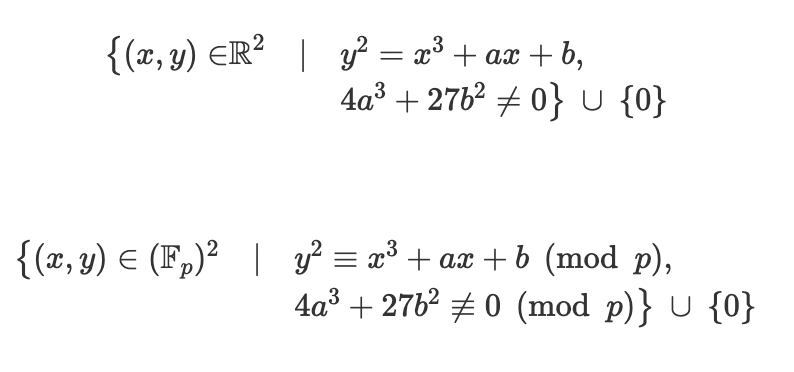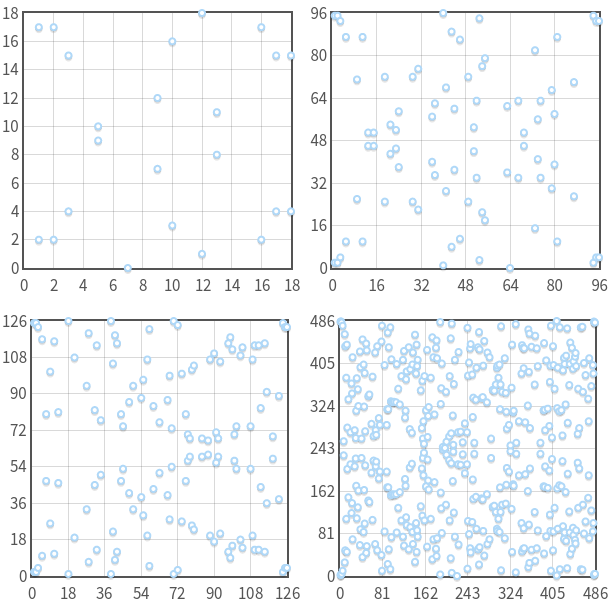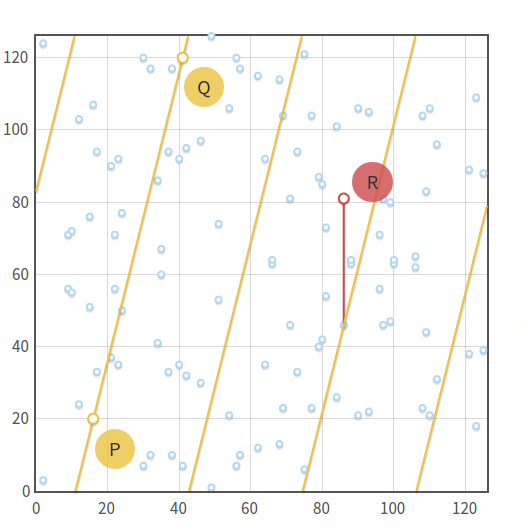• 0P = 0
• 1P = (3,6)
• 2P = (80,10)
• 3P = (80,87)
• 4P = (3, 91)
• 5P = 0
• 6P = (3, 6)
• 7P = (80, 10)

`k * P = (k mod 5) * P`

`n * P + m * P = (m + n) * P`

`n(hP) = 0`

# ECC

ECC(Elliptic Curve Cryptography)即椭圆曲线加密算法。在上文中我们说了，在有限域中的椭圆曲线乘法(指数)是相对容易计算的，但是除法(对数)则很难计算，这也是椭圆曲线得以实现非对称加密的难题假设和理论基础。

• p：定义了有限域大小的素数
• a、b：定义椭圆曲线的特征参数
• G：生成循环子群的基点
• n：循环子群的阶(order)
• h：循环子群的余因子(cofactor)

• 私钥：一个在{1, …, n - 1}范围内的随机数d
• 公钥：一个椭圆曲线上的点H = dG

```openssl ecparam -genkey -param_enc explicit -out priv.pem -name secp256k1
openssl pkeyutl -derive -inkey priv.pem -peerkey RecipientsPublicKey.pem -out SharedSecret.bin```

## ECDH

DH即Diffie–Hellman，是两个提出者的名字，Whitfield DiffieMartin Hellman。ECDH则为DH的其中一个实现，而DH是一个秘钥协商协议。秘钥协商问题可以简化为：如何在通信链路不安全的安全下安全交换秘钥。DH的实现有很多，但本质上也是基于某种不可逆的拆分操作，WiKi中有个比较直观的例子介绍了交换秘钥的过程：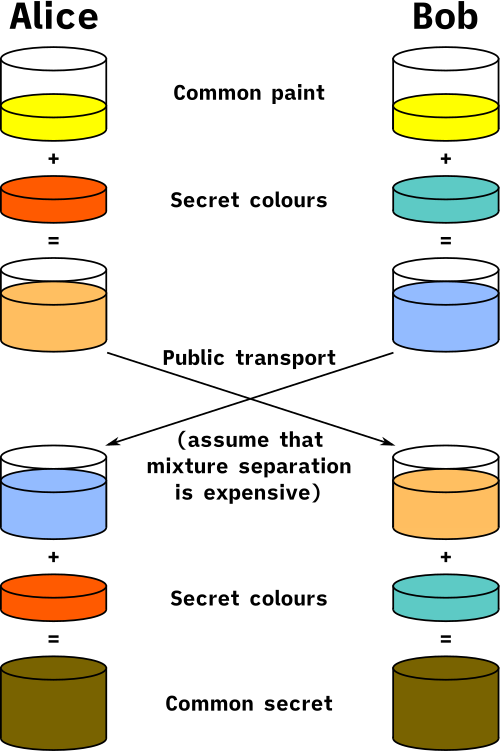1. Alice和Bob使用同样的椭圆曲线，并分别随机生成它们自己的私钥和公钥，其中Alice的私钥为da，公钥为Ha = da * G，Bob的私钥和公钥分别为db和Hb；
2. Alice和Bob在不安全的信道中交换它们的公钥Ha和Hb；
3. Alice和Bob分别计算自己私钥和收到的对方公钥的乘积，有：
`Sa = da * Hb = da * (db * G) = db * (da * G) = db * Ha = Sb`

## ECDSA

DSA(Digital Signature Algorithm)即数字签名算法。我们之前介绍RSA的时候说过RSA的签名方法，即对数据进行hash然后将其使用私钥加密，对端公钥解密并成功校验hash可认为数据没有被篡改。使用椭圆曲线实现的数字签名算法则称为ECDSA。

1. {1,…,n-1}中随机选取一个整数k
2. 计算点P = kG
3. 计算r = Px mod n (Px为点P的x坐标)
4. 如果r=0，从新选一个k
5. 计算s = k^-1 (z + r*da) mod n
6. 如果s=0，从新选一个k

Bob收到签名(r, s)后，自己也计算一份数据的哈希zHa是Alice的公钥，校验签名的过程如下：

1. 计算整数u1 = s^-1 * z mod n
2. 计算整数u1 = s^-1 * r mod n
3. 计算点P = u1 * G + u2 * Ha
4. r = Px mod n时，表示签名有效，数据未被篡改。Px为点P的x坐标

# 安全性

## 椭圆曲线的选择

`S = random() H = hash(S) a = f(H) b = g(H) ... `

`curve = EllipticCurve( 'secp256k1', # Field characteristic. p=0xfffffffffffffffffffffffffffffffffffffffffffffffffffffffefffffc2f, # Curve coefficients. a=0, b=7, # Base point. g=(0x79be667ef9dcbbac55a06295ce870b07029bfcdb2dce28d959f2815b16f81798, 0x483ada7726a3c4655da4fbfc0e1108a8fd17b448a68554199c47d08ffb10d4b8), # Subgroup order. n=0xfffffffffffffffffffffffffffffffebaaedce6af48a03bbfd25e8cd0364141, # Subgroup cofactor. h=1, ) `

ECC秘钥的长度取决于所使用的椭圆曲线，在大多数系统中，如OpenSSL、OpenSSH和比特币中默认的ECC秘钥长度都是256位。在OpenSSL中预置的曲线可见：

• https://github.com/openssl/openssl/blob/master/crypto/ec/ec_curve.c

## ECDSA中的k

1. 注意到r1 = r2，因为r = Px mod nP=kG，对两个信息是一样的
2. (s1 - s2) mod n = k^-1(z1 - z2) mod n
3. 两边同时乘以k，则 k(s1 - s2) mod n = (z1 - z2) mod n
4. 两遍同时除(s1 - s2)，则k = (z1-z2)(s1-s2)^-1 mod n

```s = k^-1(z + r * d) mod n
d = r^-1(s*k - z) mod n```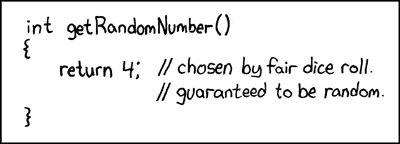## 离散对数

• Weil pairing / Tate pairing
• Semaev-Smart-Satoh-Araki attack
• Gaudry、Hess、Smart等提出的针对二进制域的度为小约数时的一种求解方法

# NSA后门

1. Hash_DRBG：基于hash函数
2. HMAC_DRBG：基于HMAC
3. CTR_DRBG：基于块加密
4. Dual_EC_DRBG：基于椭圆曲线加密

2015年，NIST发布新版本的标准，默默地去掉了Dual_EC_DRBG。

#### 留言：Process: 0.0327s ( Load:0.0003s Init:0.0008s Exec:0.0194s Template:0.0123s ) | DB :14 queries 1 writes | UseMem:3,072 kb